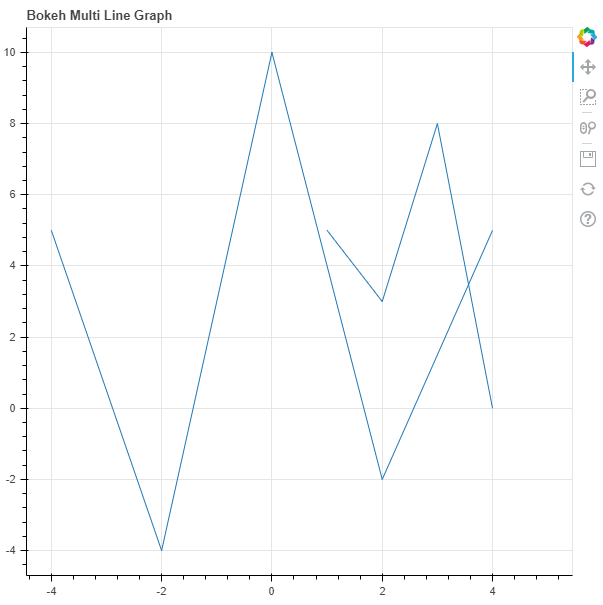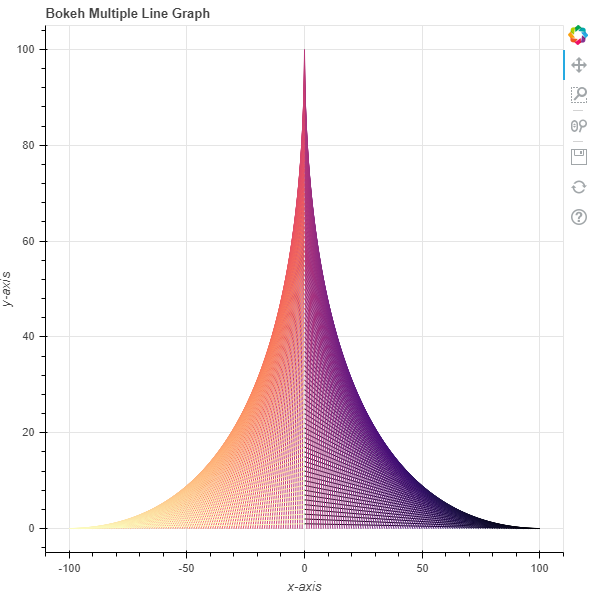# Python Bokeh – Plotting Multiple Lines on a Graph

Bokeh is a Python interactive data visualization. It renders its plots using HTML and JavaScript. It targets modern web browsers for presentation providing elegant, concise construction of novel graphics with high-performance interactivity.

Bokeh can be used to plot multiple lines on a graph. Plotting multiple lines on a graph can be done using the `multi_line()` method of the `plotting` module.

## plotting.figure.multi_line()

Syntax : multi_line(parameters)

Parameters :

• xs : x-coordinates of the lines
• ys : y-coordinates of the lines
• line_alpha : percentage value of line alpha, default is 1
• line_cap : value of line cap for the line, default is butt
• line_color : color of the line, default is black
• line_dash : value of line dash such as : solid, dashed, dotted, dotdash, dashdot [default is solid ]
• line_dash_offset : value of line dash offset, default is 0
• line_join : value of line join, default in bevel
• line_width : value of the width of the line, default is 1
• name : user-supplied name for the model
• tags : user-supplied values for the model

Other Parameters :

• alpha : sets all alpha keyword arguments at once
• color : sets all color keyword arguments at once
• legend_field : name of a column in the data source that should be used
• legend_group : name of a column in the data source that should be used
• legend_label : labels the legend entry
• muted : determines whether the glyph should be rendered as muted or not, default is False
• name : optional user-supplied name to attach to the renderer
• source : user-supplied data source
• view : view for filtering the data source
• visible : determines whether the glyph should be rendered or not, default is True
• x_range_name : name of an extra range to use for mapping x-coordinates
• y_range_name : name of an extra range to use for mapping y-coordinates
• level : specifies the render level order for this glyph

Returns : an object of class `GlyphRenderer`

Example 1 : In this example we will be using the default values for plotting the graph.

 `# importing the modules  ` `from` `bokeh.plotting ``import` `figure, output_file, show  ` `      `  `# file to save the model  ` `output_file(``"gfg.html"``)  ` `      `  `# instantiating the figure object  ` `graph ``=` `figure(title ``=` `"Bokeh Multi Line Graph"``)  ` `    `  `# the points to be plotted  ` `xs ``=` `[[``1``, ``2``, ``3``, ``4``, ``5``], [``-``4``, ``-``2``, ``0``, ``2``, ``4``]]  ` `ys ``=` `[[``5``, ``3``, ``8``, ``0``], [``5``, ``-``4``, ``10``, ``-``2``, ``5``]]  ` `   `  `# plotting the graph  ` `graph.multi_line(xs, ys)  ` `    `  `# displaying the model  ` `show(graph) `

Output :Example 2 : In this example we will be plotting the multiple lines with various other parameters

 `# importing the modules  ` `from` `bokeh.plotting ``import` `figure, output_file, show  ` `from` `bokeh.palettes ``import` `magma ` ` `  `# file to save the model  ` `output_file(``"gfg.html"``)  ` `      `  `# instantiating the figure object  ` `graph ``=` `figure(title ``=` `"Bokeh Multiple Line Graph"``)  ` `    `  `# name of the x-axis  ` `graph.xaxis.axis_label ``=` `"x-axis"` `     `  `# name of the y-axis  ` `graph.yaxis.axis_label ``=` `"y-axis"` `    `  `# the points to be plotted ` `x ``=` `[n ``for` `n ``in` `range``(``-``100``, ``101``)] ` `x.reverse() ` `xs ``=` `[[n, ``0``] ``for` `n ``in` `x] ` `y1 ``=` `[n ``for` `n ``in` `range``(``1``, ``101``)] ` `y1.reverse() ` `y ``=` `[n ``for` `n ``in` `range``(``1``, ``101``)] ``+` `[``0``] ``+` `y1 ` `ys ``=` `[[``0``, n] ``for` `n ``in` `y]  ` ` `  `# color of the lines ` `line_color ``=` `magma(``201``) ` `     `  `# plotting the graph  ` `graph.multi_line(xs, ys, ` `                 ``line_color ``=` `line_color)  ` `      `  `# displaying the model  ` `show(graph) `

Output :Whether you're preparing for your first job interview or aiming to upskill in this ever-evolving tech landscape, GeeksforGeeks Courses are your key to success. We provide top-quality content at affordable prices, all geared towards accelerating your growth in a time-bound manner. Join the millions we've already empowered, and we're here to do the same for you. Don't miss out - check it out now!

Previous
Next